1598034060

# Types Of Activation Functions used in Neural Network?

## Note

In this article I have discussed the various types of activation functions and what are the types of problems one might encounter while using each of them.

I would suggest to begin with a ReLU function and explore other functions as you move further. You can also design your own activation functions giving a non-linearity component to your network.

Recall that inputs x0,x1,x2,x3……xn and weights w0,w1,w2,w3………wn are multiplied and added with bias term to form our input.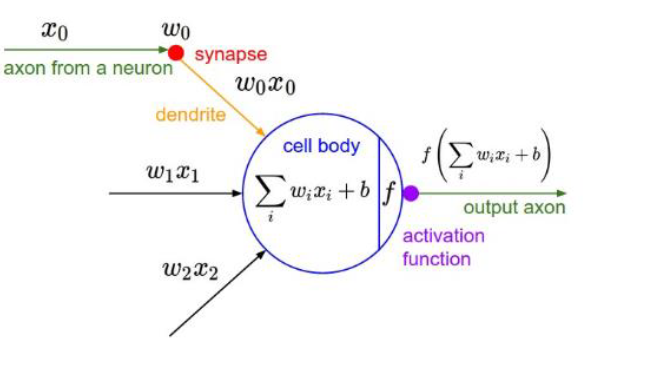Clearly W implies how much weight or strength we want to give our incoming input and we can think** b** as an offset value, making x*w have to reach an offset value before having an effect.

### As far we have seen the inputs so now what is activation function?

Activation function is used to set the boundaries for the overall output value.For Example:-let **z=X*w+b **be the output of the previous layer then it will be sent to the activation function for limit it’svalue between 0 and 1(if binary classification problem).

Finally, the output from the activation function moves to the next hidden layer and the same process is repeated. This forward movement of information is known as the forward propagation.

What if the output generated is far away from the actual value? Using the output from the forward propagation, error is calculated. Based on this error value, the weights and biases of the neurons are updated. This process is known as back-propagation.

#activation-functions #softmax #sigmoid-function #neural-networks #relu #function

## Buddha Community1598034060

## Note

In this article I have discussed the various types of activation functions and what are the types of problems one might encounter while using each of them.

I would suggest to begin with a ReLU function and explore other functions as you move further. You can also design your own activation functions giving a non-linearity component to your network.

Recall that inputs x0,x1,x2,x3……xn and weights w0,w1,w2,w3………wn are multiplied and added with bias term to form our input.Clearly W implies how much weight or strength we want to give our incoming input and we can think** b** as an offset value, making x*w have to reach an offset value before having an effect.

### As far we have seen the inputs so now what is activation function?

Activation function is used to set the boundaries for the overall output value.For Example:-let **z=X*w+b **be the output of the previous layer then it will be sent to the activation function for limit it’svalue between 0 and 1(if binary classification problem).

Finally, the output from the activation function moves to the next hidden layer and the same process is repeated. This forward movement of information is known as the forward propagation.

What if the output generated is far away from the actual value? Using the output from the forward propagation, error is calculated. Based on this error value, the weights and biases of the neurons are updated. This process is known as back-propagation.

#activation-functions #softmax #sigmoid-function #neural-networks #relu #function1596927420

## Activation Functions in Neural Network

Activation function, as name suggests, decides whether a neuron should be activated or not based on addition of a bias with the weighted sum of inputs. Hence, it is a very significant component of Deep Learning, as they in a way determine the output of models. Activation function has to be efficient so the model can scale along the increase in number of neurons.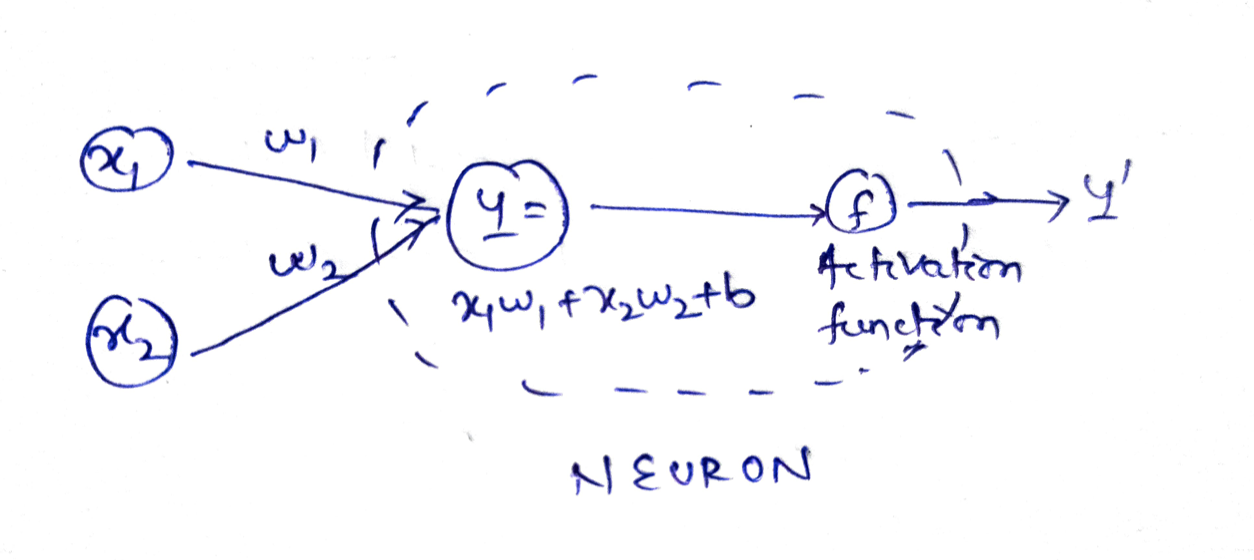To be precise, activation function decides how much information of the input relevant for the next stage.

For example, suppose x1 and x2 are two inputs with w1 and w2 their respective weights to the neuron. The output Y = activation_function(y).

Here, y = x1.w1 + x2.w2 + b i.e. weighted sum of inputs and bias.

Activation functions are mainly of 3 types. We will analyse the curves, pros and cons of each here. The input we work on will be a arithmetic progression in [-10, 10] with a constant difference of 0.1

``````x = tf.Variable(tf.range(-10, 10, 0.1), dtype=tf.float32)
``````

### Binary step

A binary step function is a threshold based activation function. If the input value is above or below a certain threshold, the neuron is activated and sends exactly the same signal to the next layer.

``````#Binary Step Activation
def binary_step(x):
return np.array([1 if each > 0 else 0 for each in list(x.numpy())])
do_plot(x.numpy(), binary_step(x), 'Binary Step')
``````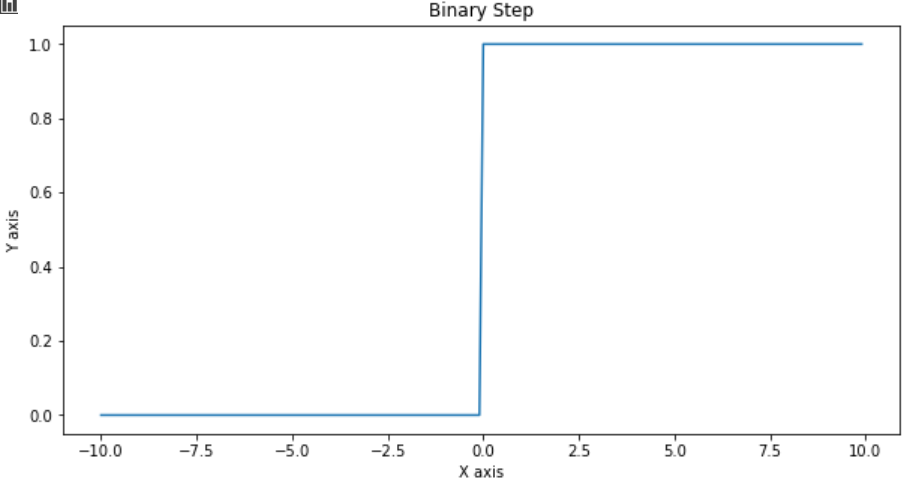Binary step is not used mostly because of two reasons. Firstly it allows only 2 outputs that doesn’t work for multi-class problems. Also, it doesn’t have a derivative.

### Linear

As the name suggests, the output is a linear function of the input i.e. y = cx

``````#Linear Activation
def linear_activation(x):
c = 0.1
return c*x.numpy()
do_plot(x.numpy(), linear_activation(x), 'Linear Activation')
``````

#activation-functions #artificial-intelligence #neural-networks #deep-learning #data-science #function1599233280

## Activation functions in Neural Networks

### What are Activation Functions?

Activation functions in neural networks are used to define the output of the neuron given the set of inputs. These are applied to the weighted sum of the inputs and transform them into output depending on the type of activation used.

Output of neuron = Activation(weighted sum of inputs + bias)

### Why we need Activation Functions?

The main idea behind using activation functions is to add non-linearity.

Now, the question arises why we need non-linearity? We need neural network models to **learn and represent complex functions. **Thus, using activation functions in neural networks, aids in process of learning complex patterns from data and adds the capability to generate non-linear mappings from inputs to outputs.

### Types of Activation Functions

1. Sigmoid- It limits the input value between 0 and 1.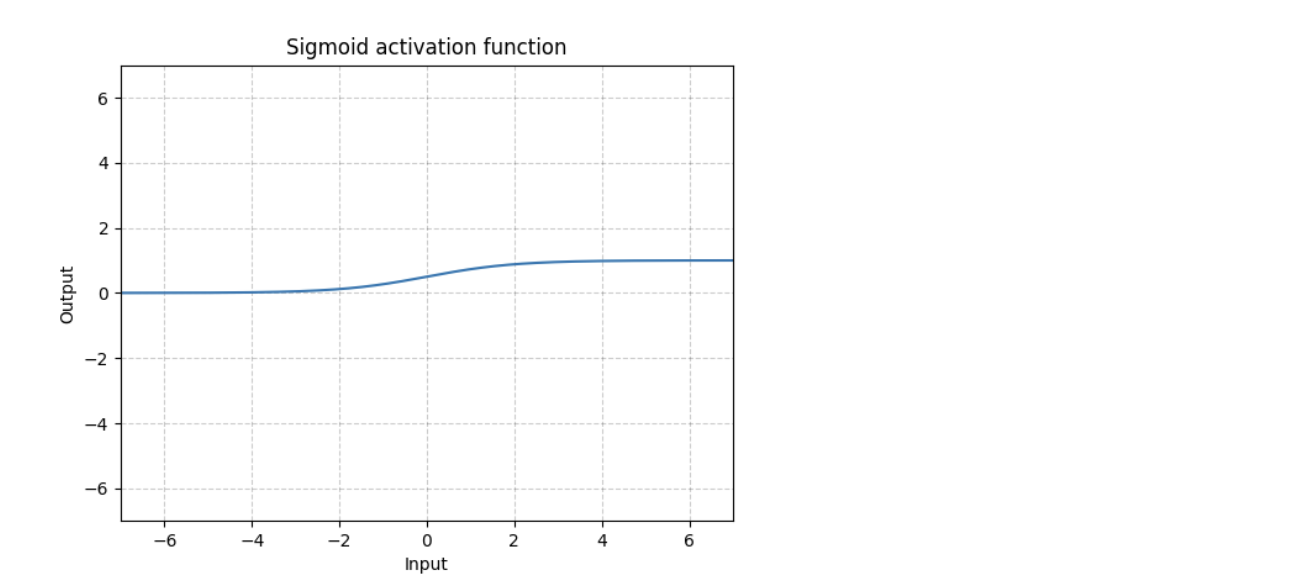Sigmoid maps the input to a small range of [0, 1]. As a result, there are large regions of the input space which are mapped to a very small range. This leads to a problem called vanishing gradient. It means that the most upstream layers will learn very slowly because essentially the computed gradient is very small due to the way the gradients are chained together.

2. Tanh- It limits the value between -1 and 1.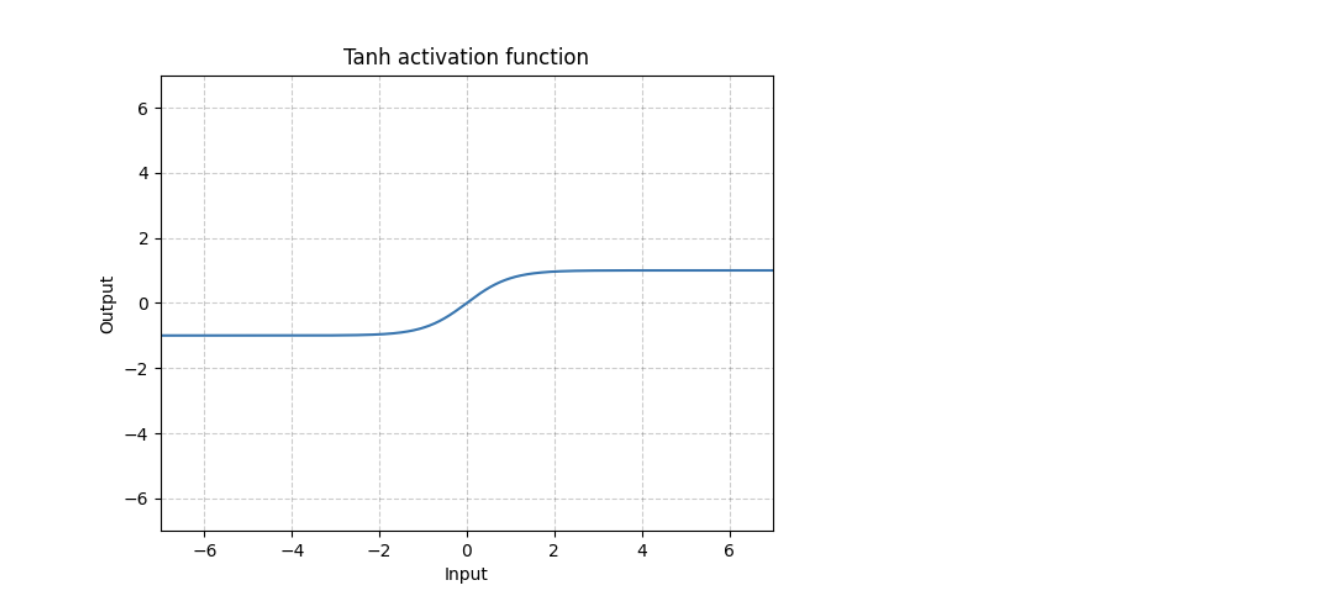**Difference between tanh and sigmoid **— Apart from the difference in the range of these activation functions, tanh function is symmetric around the origin, whereas the sigmoid function is not.

Both sigmoid and tanh pose vanishing gradient problems when used as activation functions in neural networks.

**3. ReLU(Rectified Linear Unit)- **It is the most popular activation function.• Outputs the same value for a positive value and zeroes out negative values.
• It is very fast to compute (given the simplicity of logic), thus improving training time.
• ReLU does not pose vanishing gradient problem.
• It does not have a maximum value.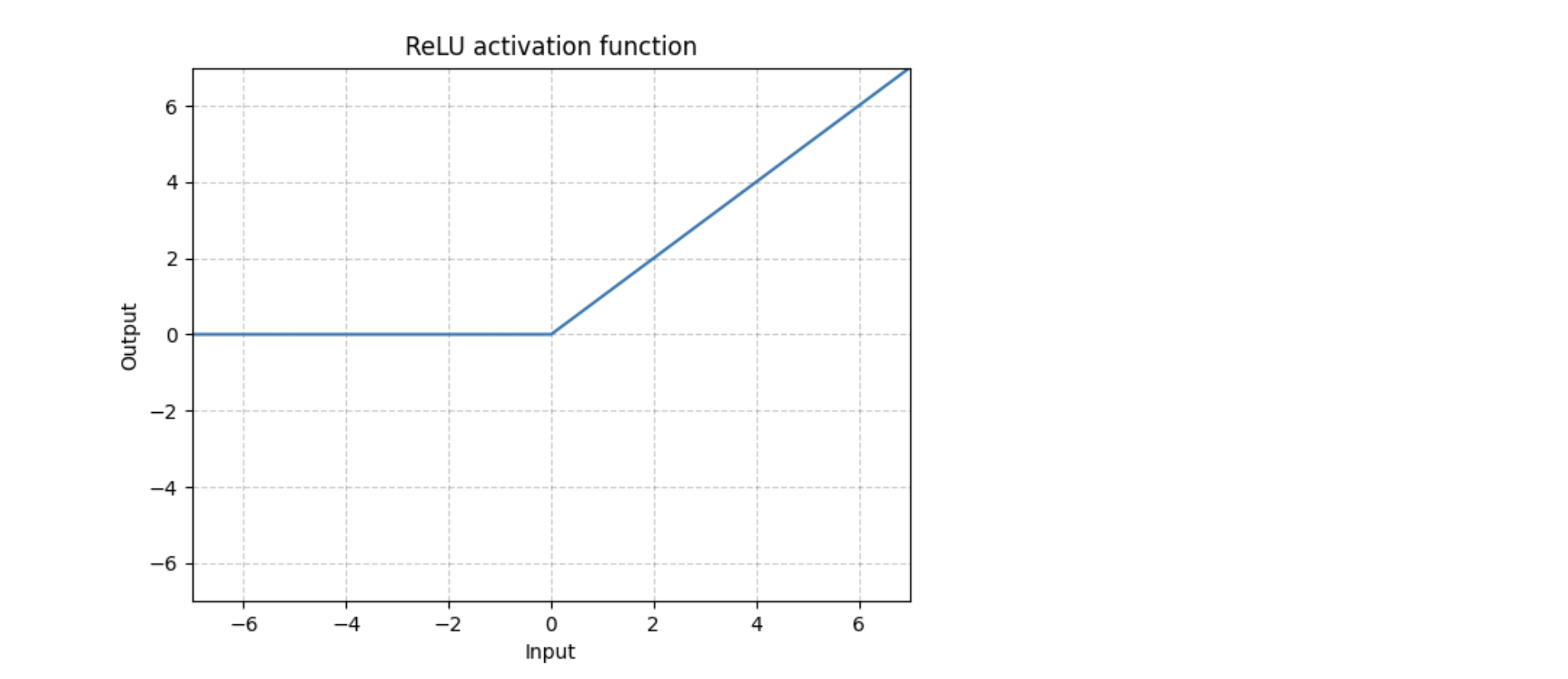There are different variations of ReLU that are available like LeakyReLU, SELU, ELU, SReLU. Still, ReLU is widely used as it is simple, fast, and efficient

#neural-networks #activation-functions #deep-learning #convolutional-network #relu1595579340

## Types of Activation Functions in Neural Network

What is an Activation Function?

The activation function is usually an abstraction representing the rate of action potential firing in the cell. In its simplest form, this function is binary — that is, either the neuron is firing or not.

What is the role of activation function in neural network?

The goal of the activation function is to introduce non-linearity into the output of a neuron.

Why do we need Non-linear activation functions ?

If you were to use linear activation functions (identity activation functions, eg: y=ax), then the neural network is just outputting a linear function of the input. In other words, no matter how many layers the network has, it will behave just like a single-layer perceptron, because summing these layers would give you just another linear function.

Types of Activation Function:

There are many types of activation functions. In this article, we are going to see the functions that I used in my projects along with python implementation for each function.

1. Sigmoid
2. Tanh
3. Relu
4. Selu
5. Softplus
6. Softsign

Sigmoid Function:

We are familiar with this function as we have used this in logistic regression.

**Mathematical Equation : f(x)= **1/(1+e^(-x))

The value range is from 0 to 1.

Python Implementation: Kindly refer the Github link “https://github.com/vivekpandian08/activation-function “

Tanh Function:

Tangent Hyperbolic function. It gives better results than using sigmoidal function but not the best.

**Mathematical Equation : f(x)= **(2/(1 + e-2x))-1

The value range is from -1 to 1.

Relu Function:

Rectified linear unit. It is the most used activation function in the hidden units for its not linear nature.

#neural-networks #activation-functions #data-science #deep #deep learning1599214020

## NEURAL NETWORK - ACTIVATION FUNCTION

In neural network activation function are used to determine the output of that neural network.This type of functions are attached to each neuron and determine whether that neuron should activate or not, based on each neuron’s input is relevant for the model’s prediction or not.In this article we going to learn different types of activation function and their advantages ,disadvantages.

Before going to study activation function let’s see how activation function works.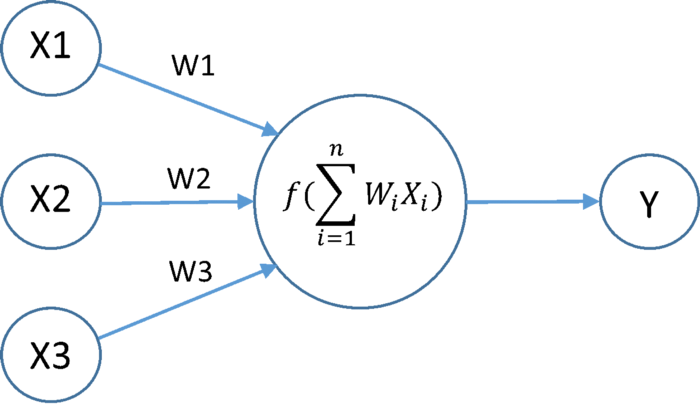fig1.Activation function

we know that each neuron contain activation function .It takes input as summation of product of outputs of previous layer with respective their weights.This summation value is passed to activation function.

### 1. Sigmoid Function:-

Sigmoid function is a one of the most popular activation function.Equation of sigmoid function reprsented as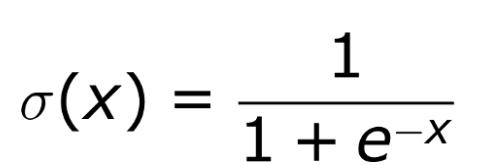sigmoid function always gives output in range of (0,1) .The derivative of sigmoid function is f`(x) =f(x)(1-f(x)) and it’s range between (0,0.25).

Generally sigmoid function is used in end layers.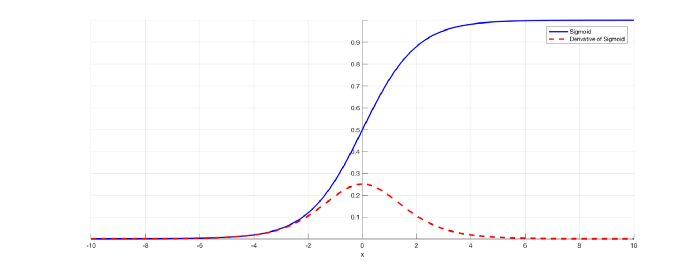sigmoid activation function

1 Smooth gradient, preventing “jumps” in output values.

2 Output values bound between 0 and 1, normalizing the output of each neuron.

1 Not a zero centric function.

3 Output of values which are far away from centroid is close to zero.

4 Computationally expensive because it has to calculate exponential value in function.

### 2 Tanh or Hyperbolic tangent activation function:-

To overcome disadvantage of non-zero centric function of sigmoid function people introduced tanh activation function.Tanh activation function equation and graph represented as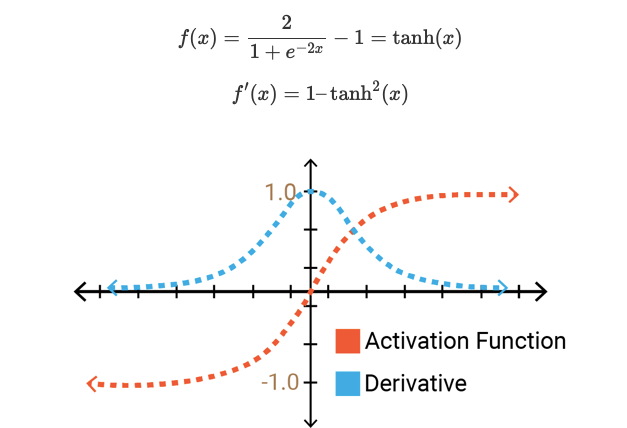Tanh activation function

the output of Tanh activation function always lies between (-1,1) and it’s derivative lies between (0,1)

Tanh function have all advantages of sigmoid function and it also a zero centric function.

1 more computation expensive than sigmoid function.

3 output of values which are far away from centroid is close to zero.

### 3 RELU(Rectified Linear Unit):-

In above two activation we have major problem with gradient vanishing to overcome this problem people introduced relu activation function.

Relu activation function is simple f(x) = max(0,x) .which means if x(input value) is positive then output also x.If x(input value) is negative then output value is zero,which means that particular neuron is deactivated.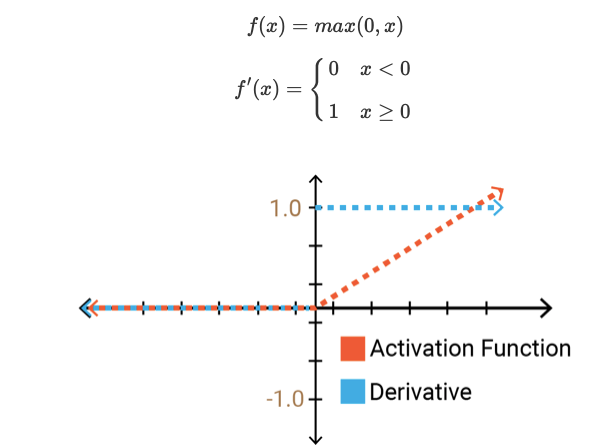RELU activation function